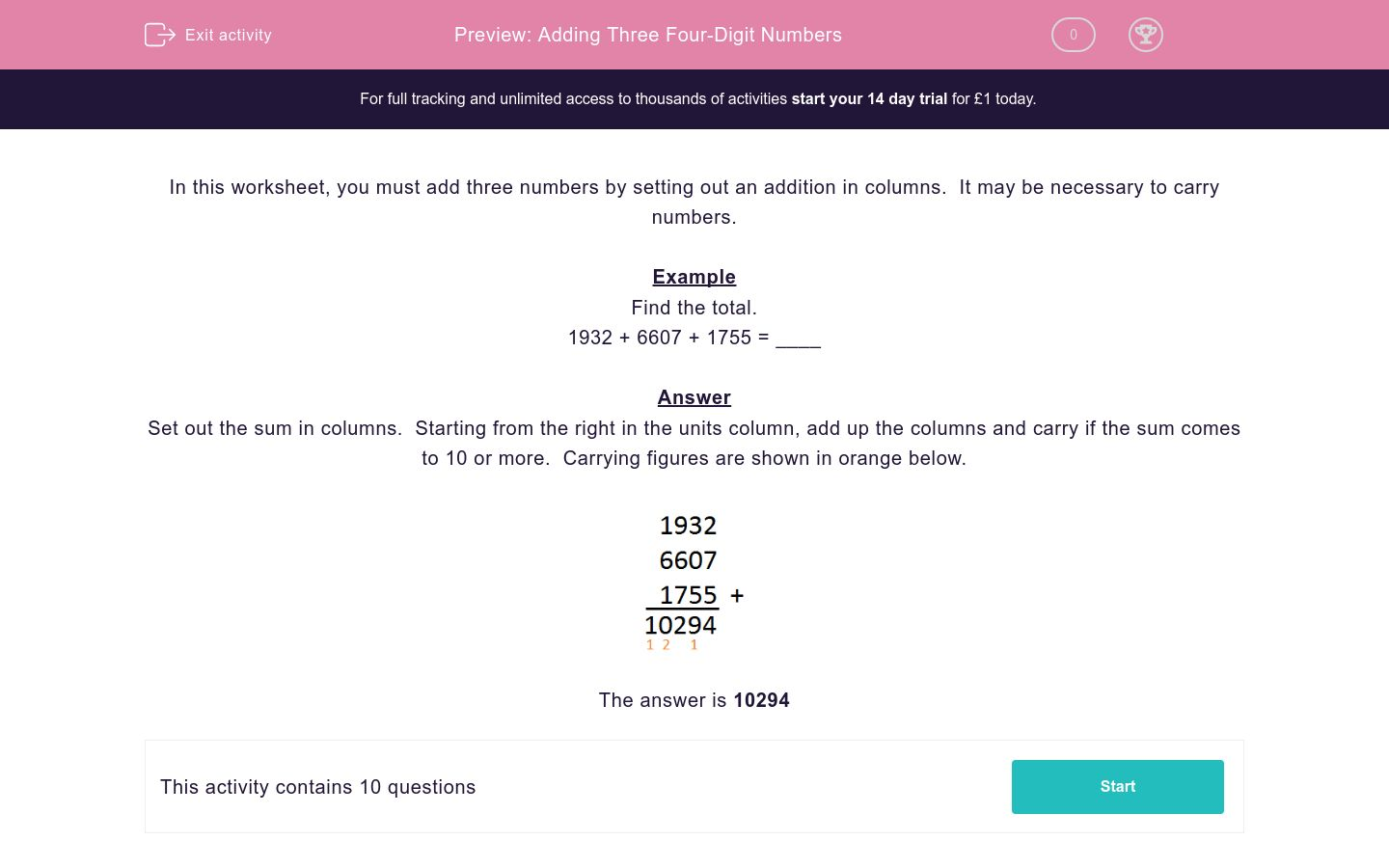### Affordable pricing from £10/month

In this worksheet, students add three 4-digit whole numbers by setting out their addition in columns. It may be necessary to carry numbers.Key stage:  KS 2

Curriculum topic:   Maths and Numerical Reasoning

Curriculum subtopic:   Mixed Problems

Difficulty level:### QUESTION 1 of 10

In this worksheet, you must add three numbers by setting out an addition in columns.  It may be necessary to carry numbers.

Example

Find the total.

1932 + 6607 + 1755 = ____

Set out the sum in columns.  Starting from the right in the units column, add up the columns and carry if the sum comes to 10 or more.  Carrying figures are shown in orange below.Find the total.

6724 + 5626 + 5332 = ____

Find the total.

6724 + 8183 + 5332 = ____

Find the total.

5626 + 8183 + 5332 = ____

Find the total.

6724 + 8183 + 4695 = ____

Find the total.

6724 + 5626 + 4695 = ____

Find the total.

5626 + 8183 + 4695 = ____

Find the total.

6724 + 5332 + 4695 = ____

Find the total.

5626 + 5332 + 4695 = ____

Find the total.

8183 + 5332 + 4695 = ____

Find the total.

2023 + 166 + 6607 = ____

• Question 1

Find the total.

6724 + 5626 + 5332 = ____

17682
• Question 2

Find the total.

6724 + 8183 + 5332 = ____

20239
• Question 3

Find the total.

5626 + 8183 + 5332 = ____

19141
• Question 4

Find the total.

6724 + 8183 + 4695 = ____

19602
• Question 5

Find the total.

6724 + 5626 + 4695 = ____

17045
• Question 6

Find the total.

5626 + 8183 + 4695 = ____

18504
• Question 7

Find the total.

6724 + 5332 + 4695 = ____

16751
• Question 8

Find the total.

5626 + 5332 + 4695 = ____

15653
• Question 9

Find the total.

8183 + 5332 + 4695 = ____

18210
• Question 10

Find the total.

2023 + 166 + 6607 = ____

8796
---- OR ----

Sign up for a £1 trial so you can track and measure your child's progress on this activity.

### What is EdPlace?

We're your National Curriculum aligned online education content provider helping each child succeed in English, maths and science from year 1 to GCSE. With an EdPlace account you’ll be able to track and measure progress, helping each child achieve their best. We build confidence and attainment by personalising each child’s learning at a level that suits them.

Get started